# 误差分析

## 模型误差原因分析

1. 如果模型在训练集的准确率就不好，说明模型欠拟合，应该重新调整模型，提高训练集的拟合效果；
2. 如果模型在训练集的准确率高而在测试集的准确率很低，就说明了模型存在过拟合的问题。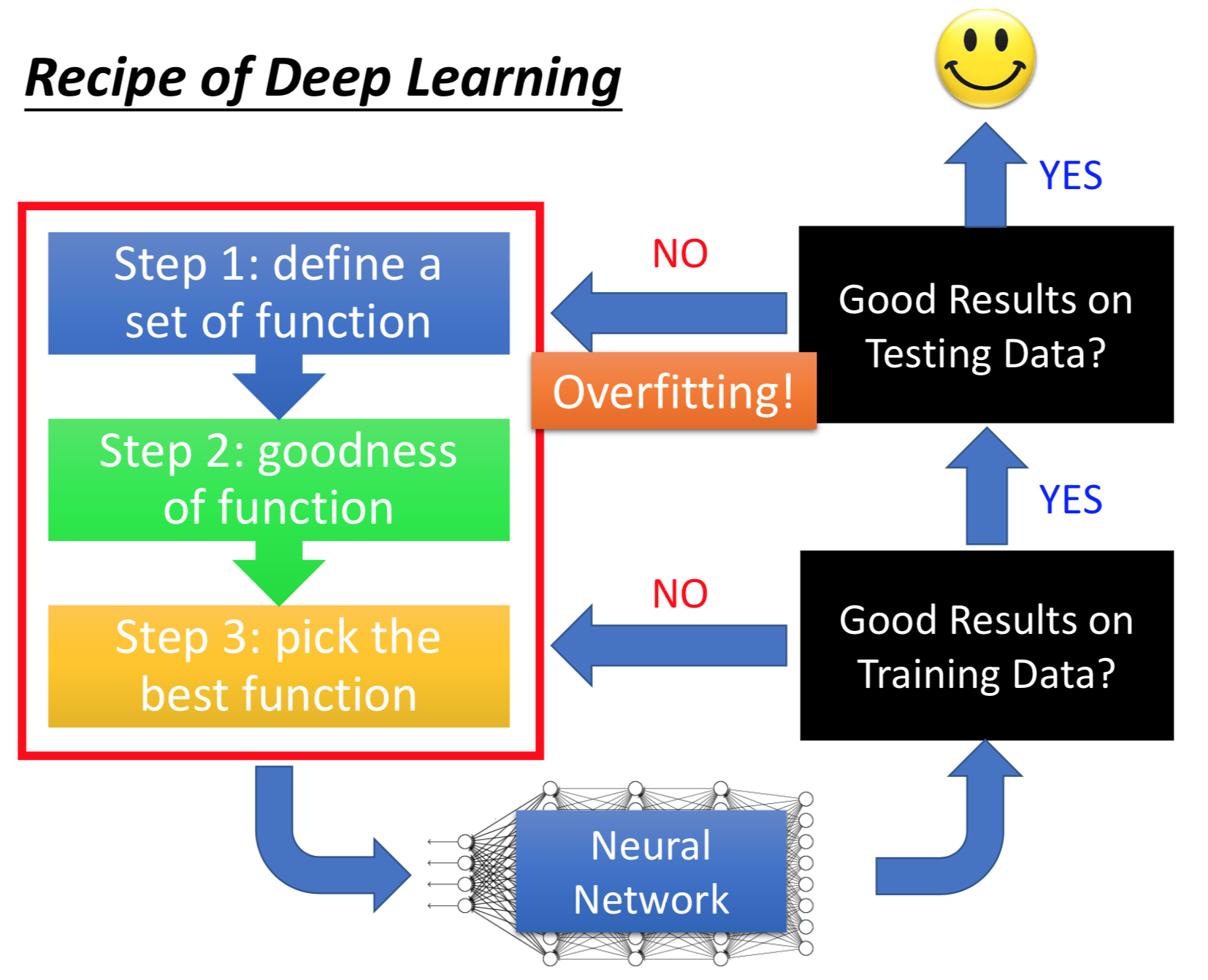$\phantom{\rule{1em}{0ex}}$$\quad$

## 模型优化方案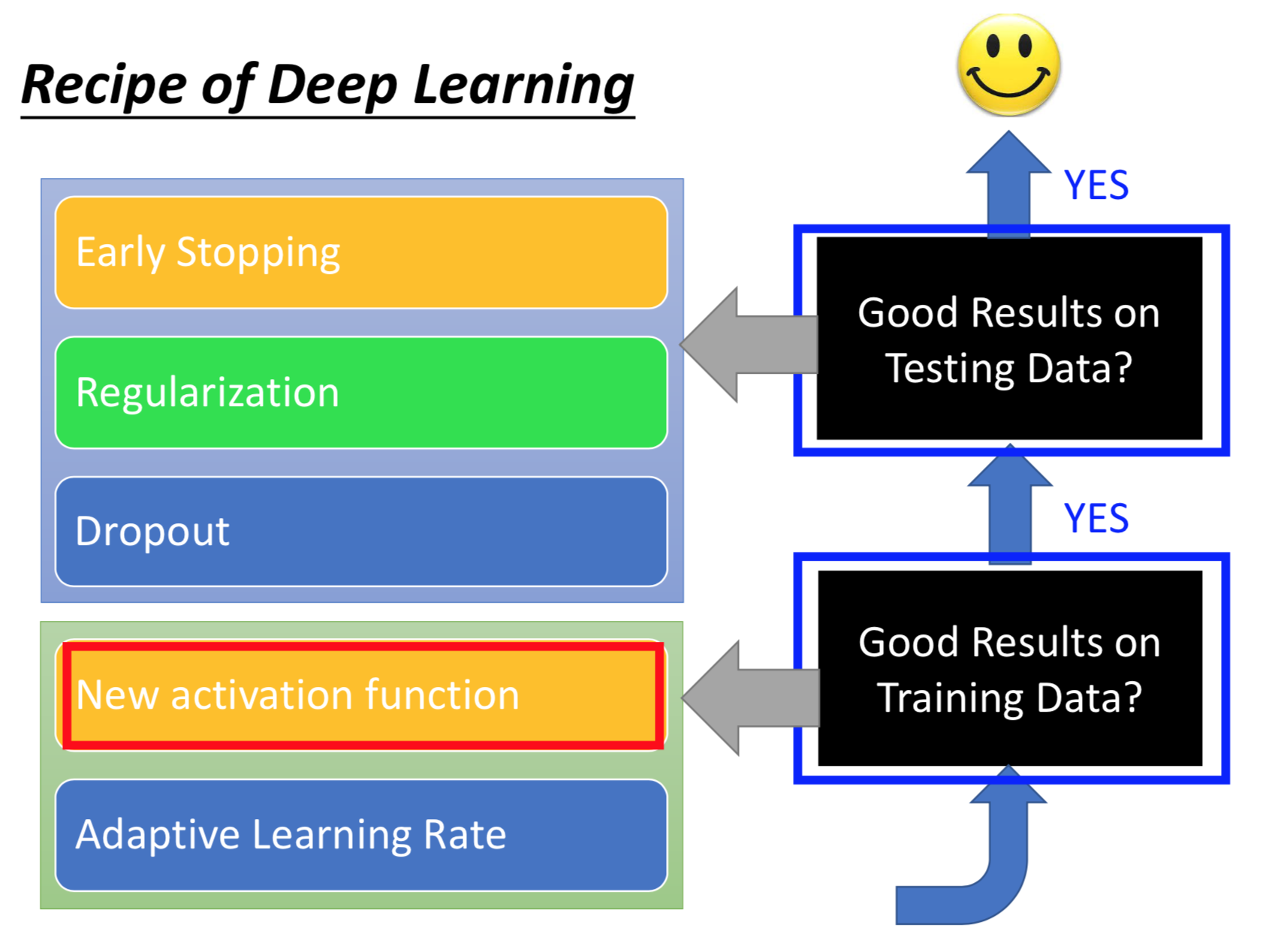$\phantom{\rule{1em}{0ex}}$$\quad$

# New activation function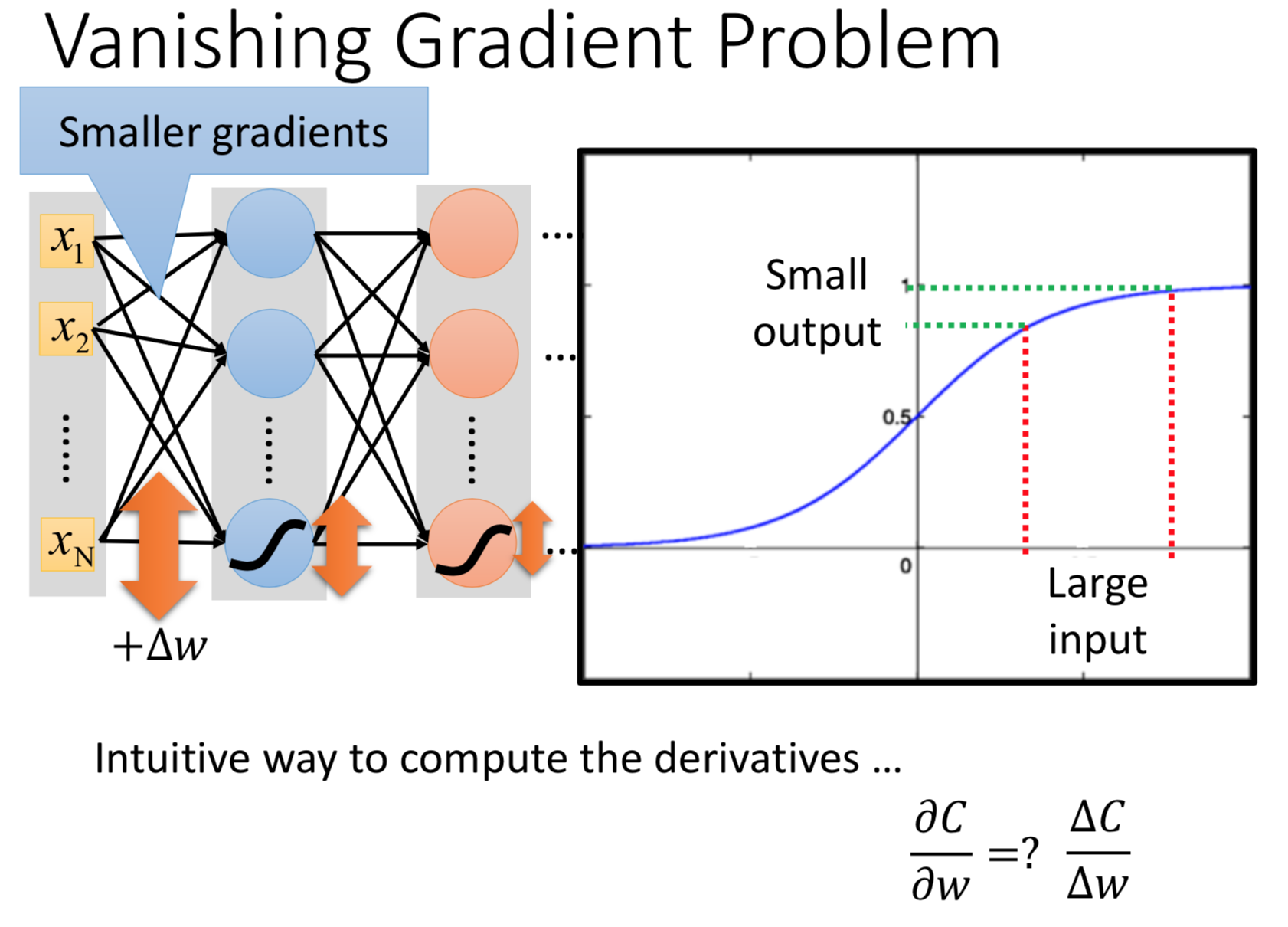$\phantom{\rule{1em}{0ex}}$$\quad$

## ReLU

ReLU全称Rectified Linear Unit，以ReLU作为激活函数可以解决梯度消失问题，通过ReLU对于一个样本通常整个神经网络被简化为较简单的形式（剔除为output为0的神经元）。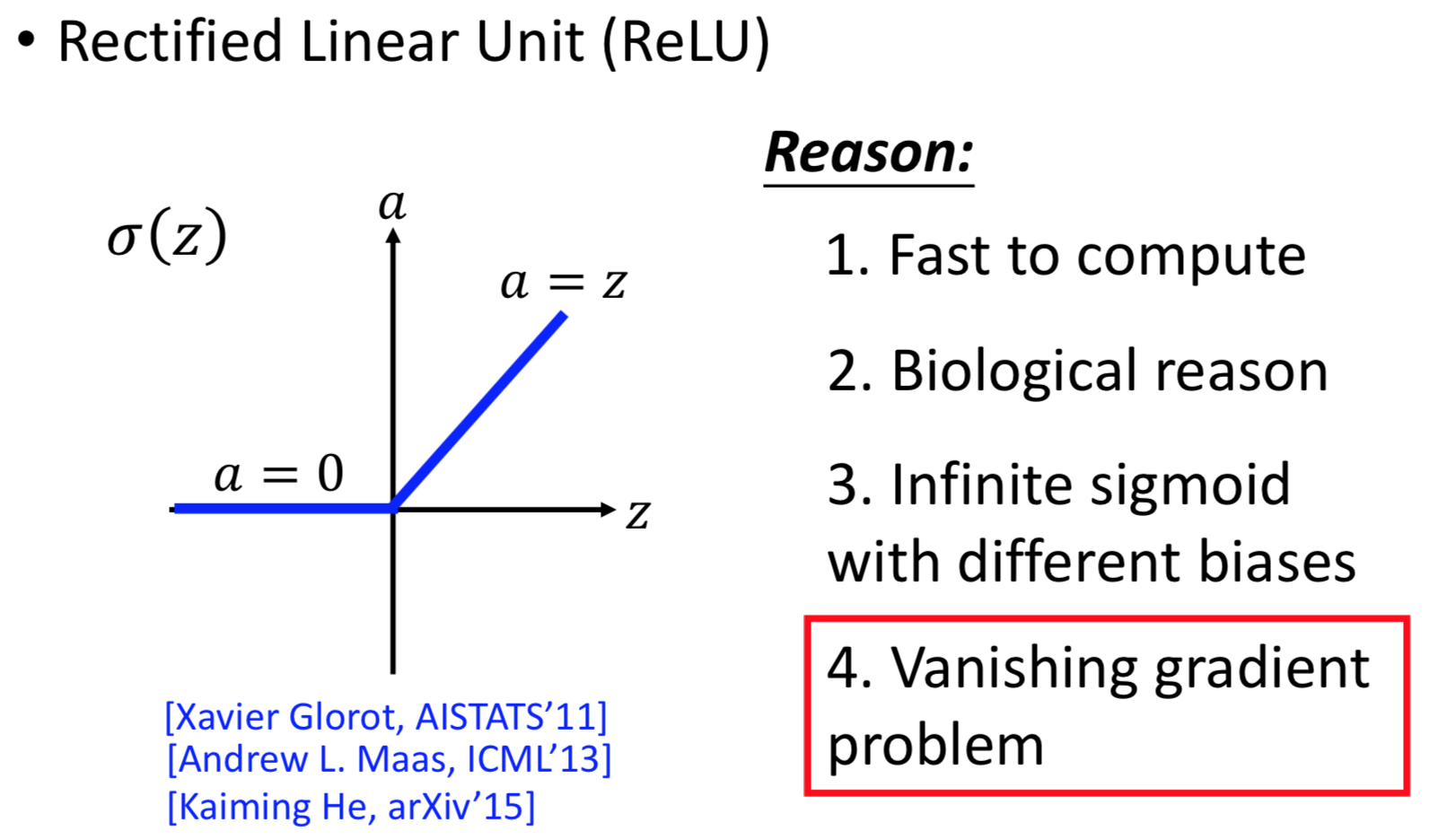ReLU还有多种变形：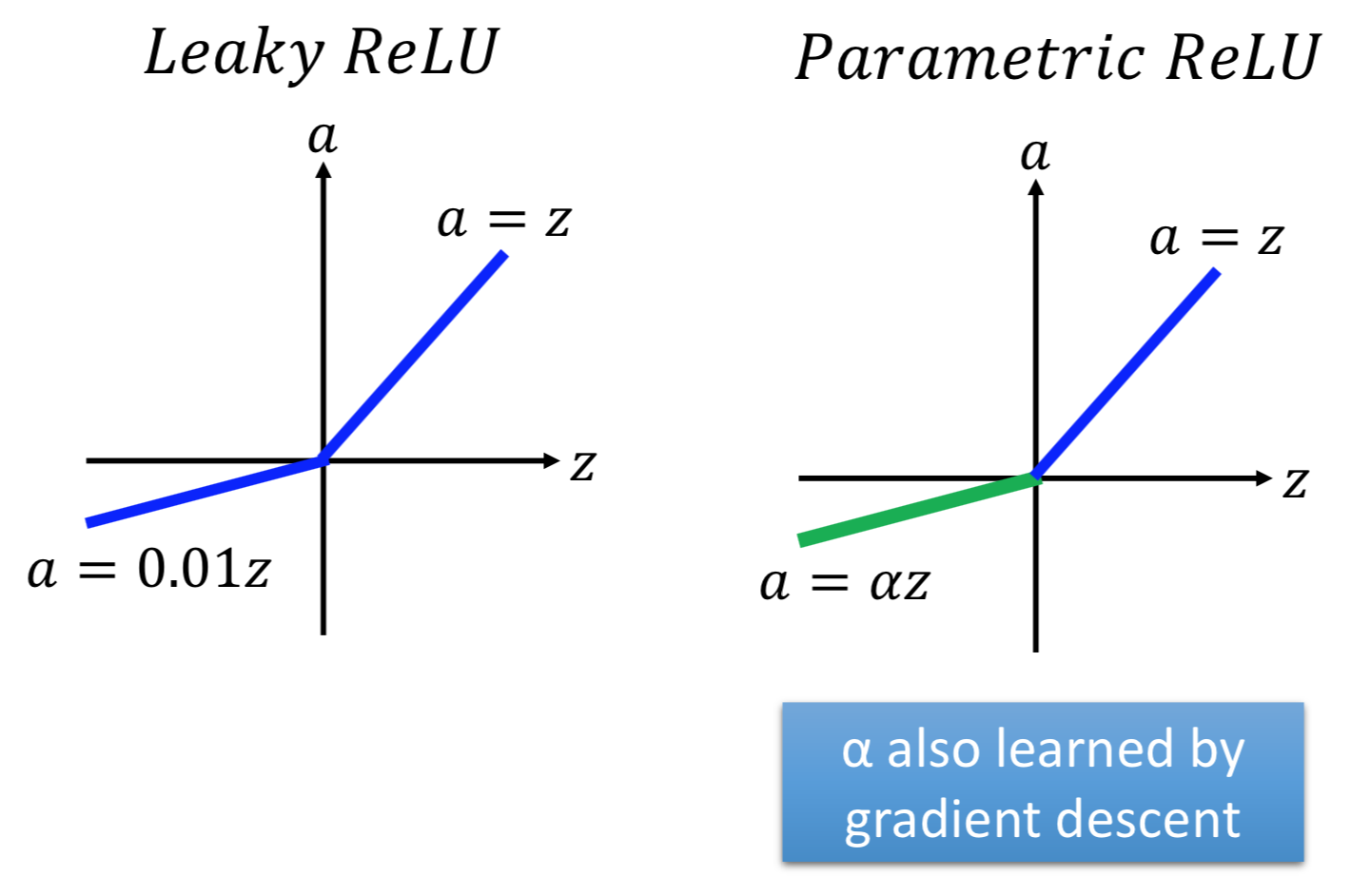$\phantom{\rule{1em}{0ex}}$$\quad$

## Maxout

### Maxout介绍

Maxout让神经网络自动地学习适合的激活函数，如图，$\left({x}_{1},{x}_{2}\right)$$(x_1,x_2)$作为输入向量，连接4个神经元，得到4个数值，以两个为一组，取组内最大值，再重复一次得到2、4。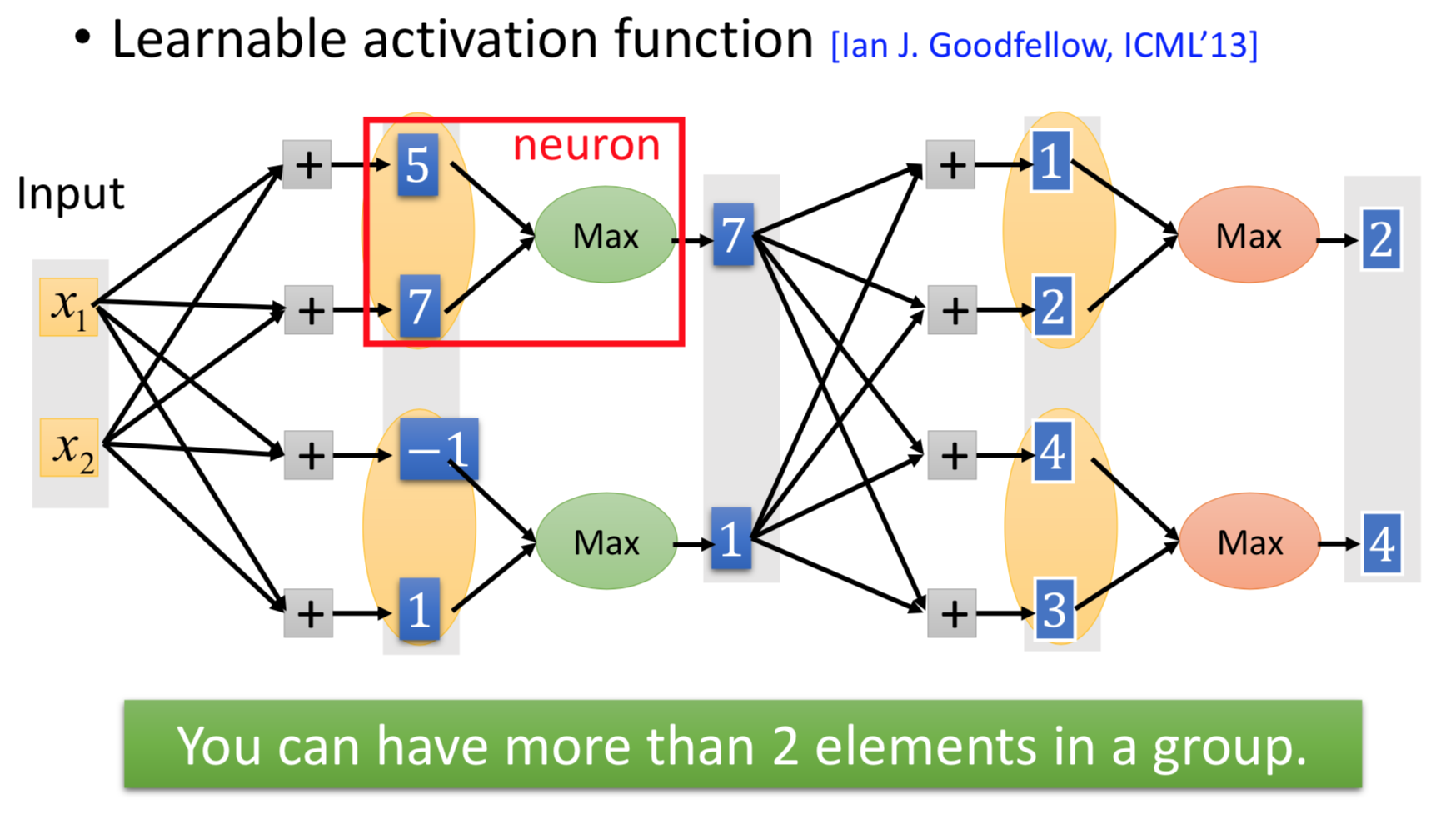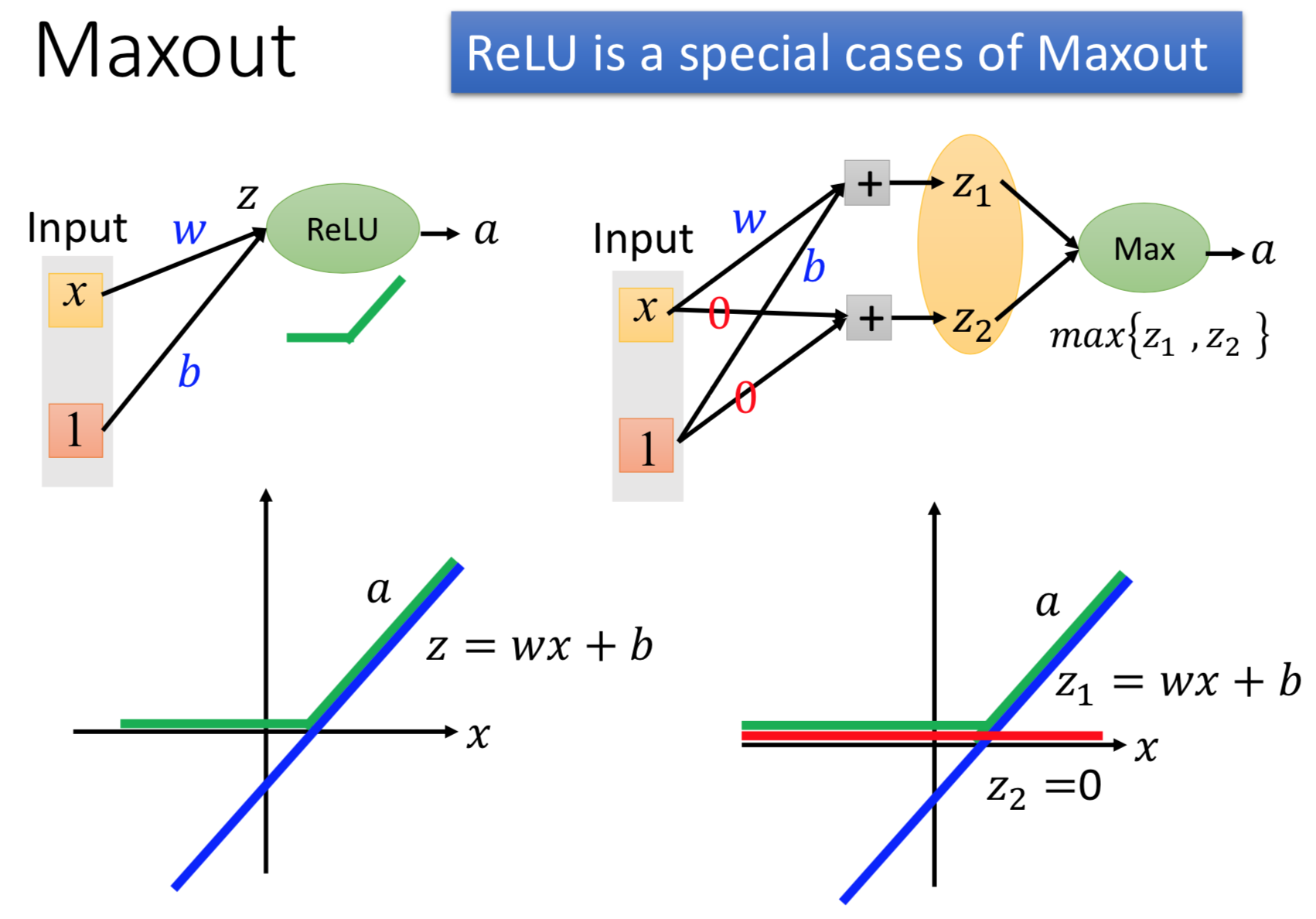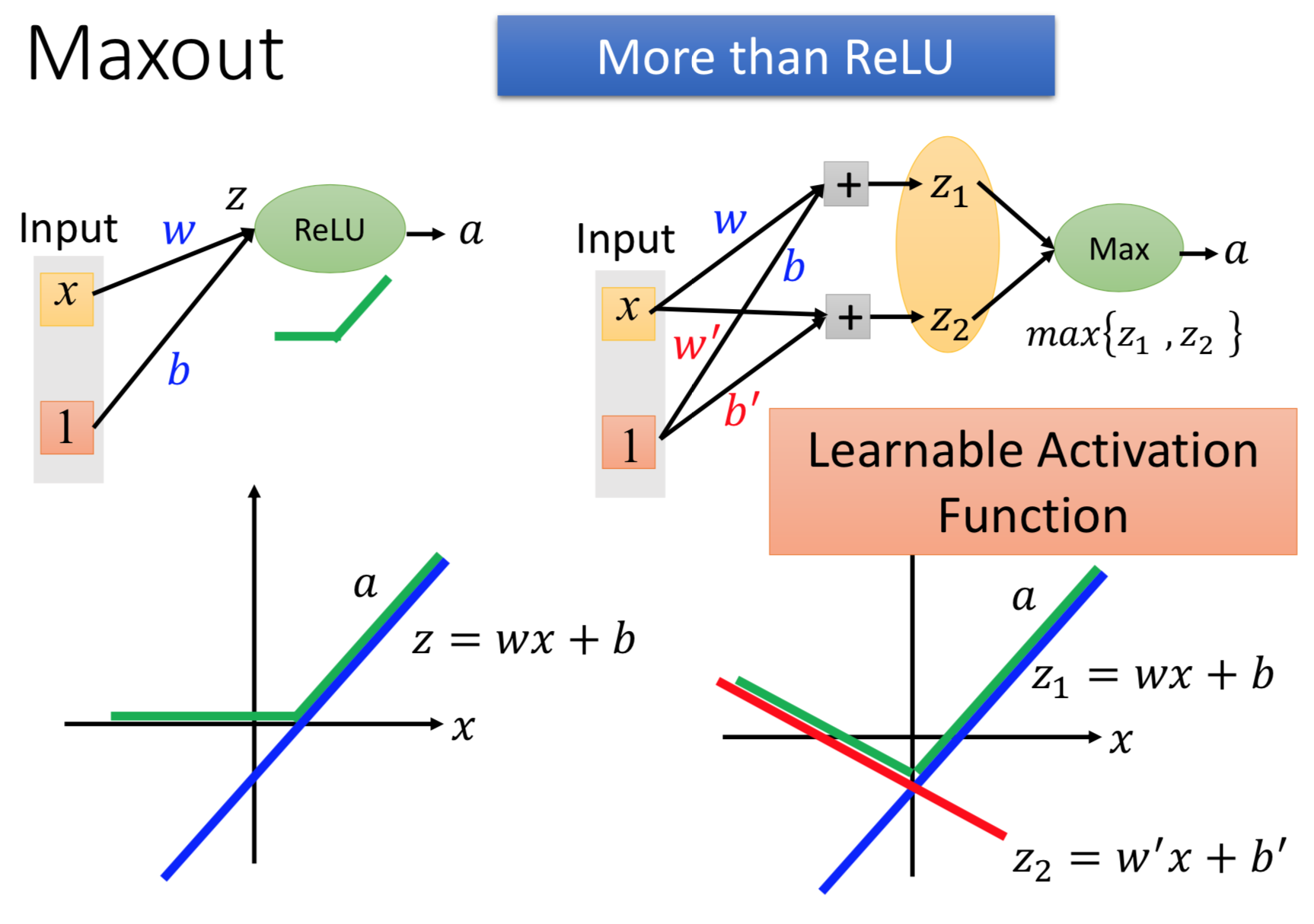$\phantom{\rule{1em}{0ex}}$$\quad$

### Maxiout方法的训练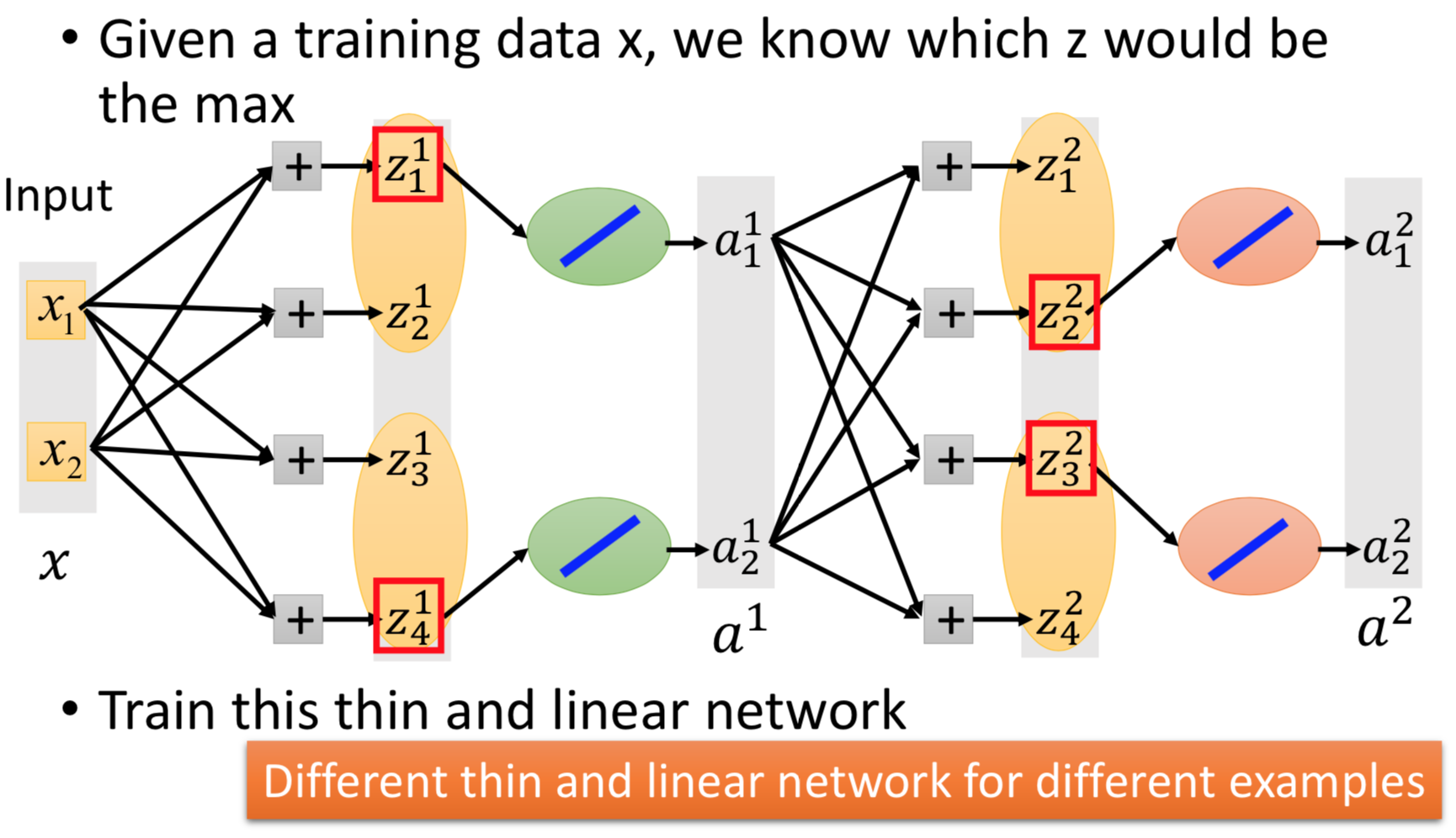$\phantom{\rule{1em}{0ex}}$$\quad$

$\phantom{\rule{1em}{0ex}}$$\quad$

## RMSProp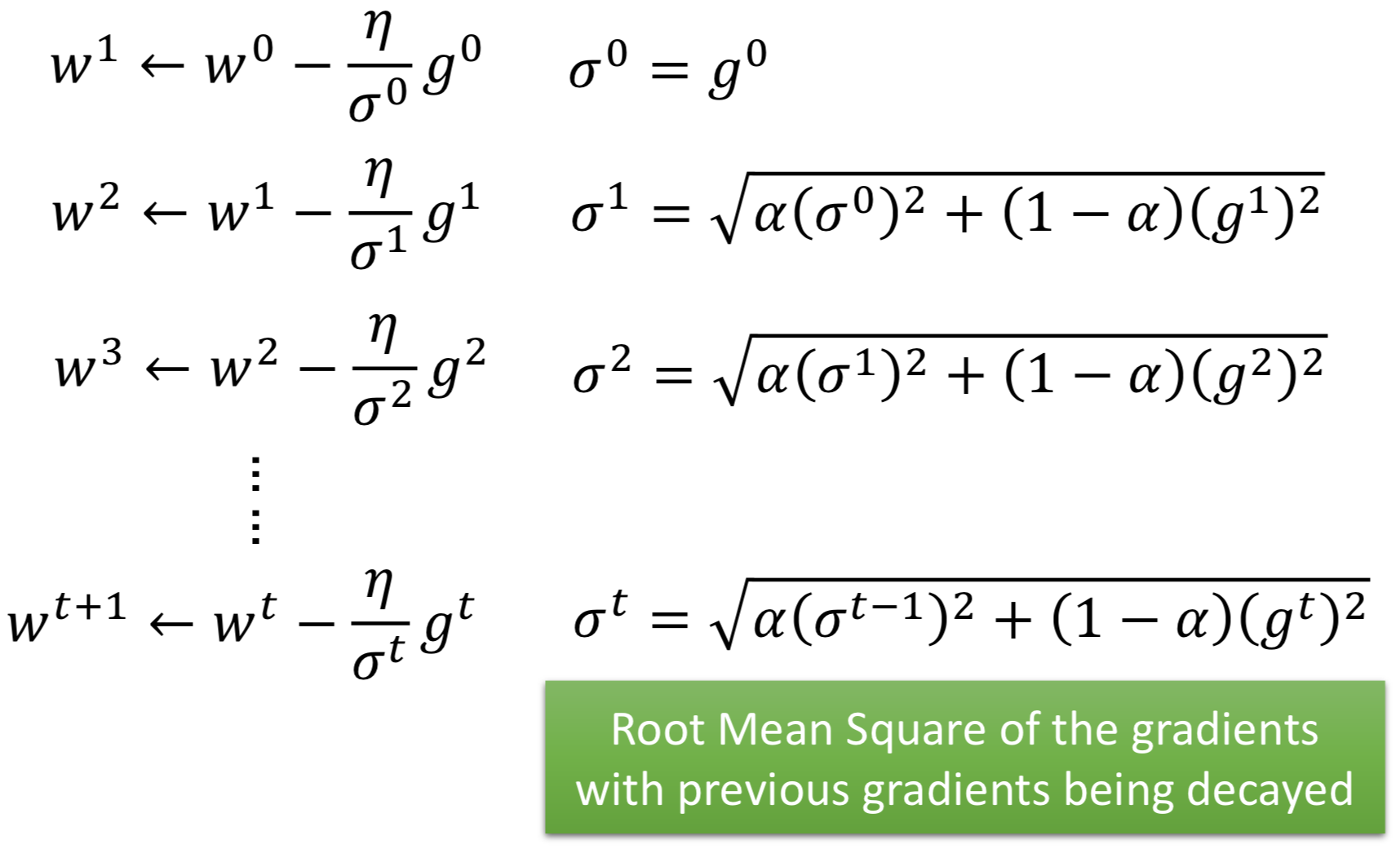$\phantom{\rule{1em}{0ex}}$$\quad$

## Momentum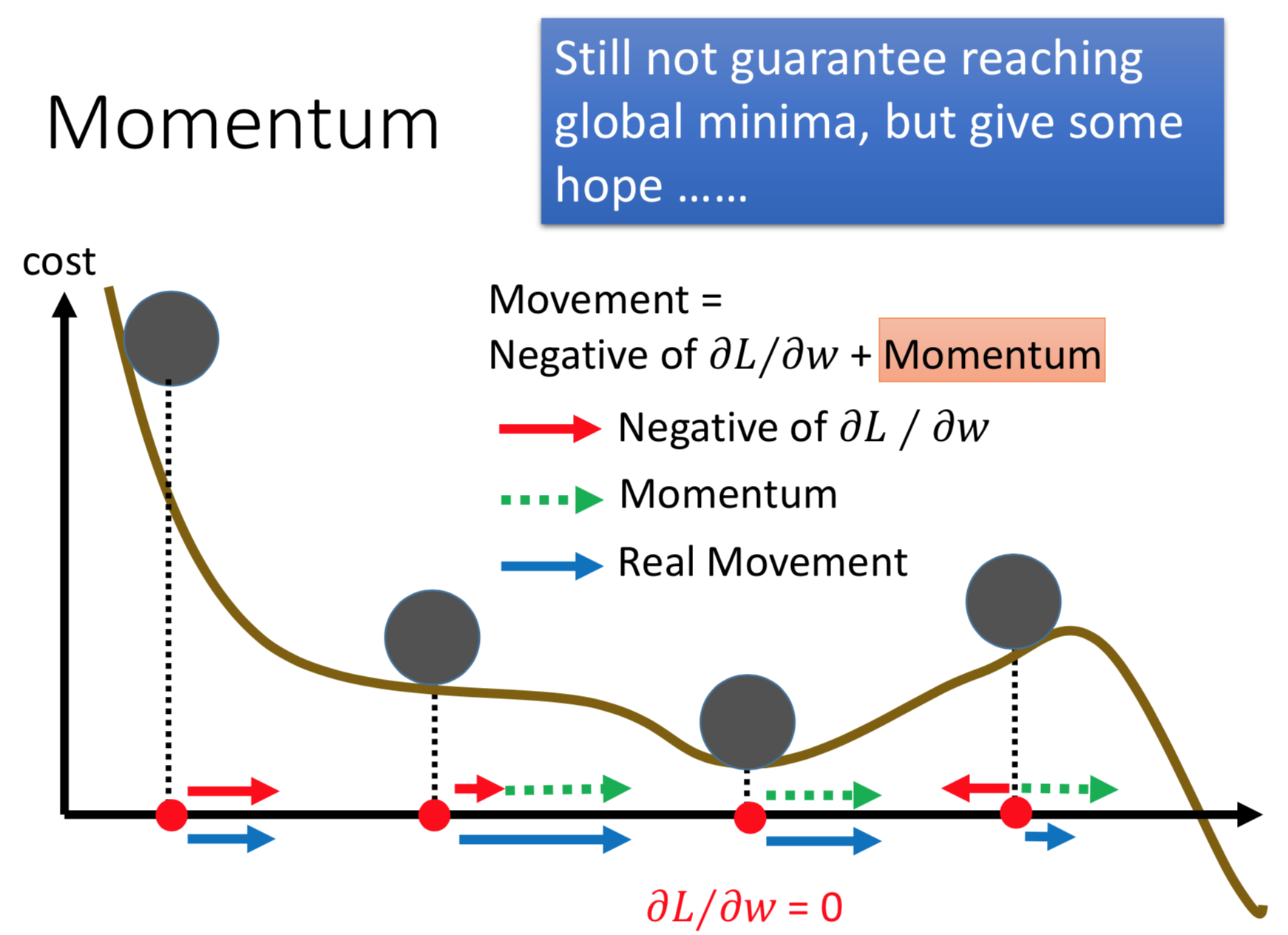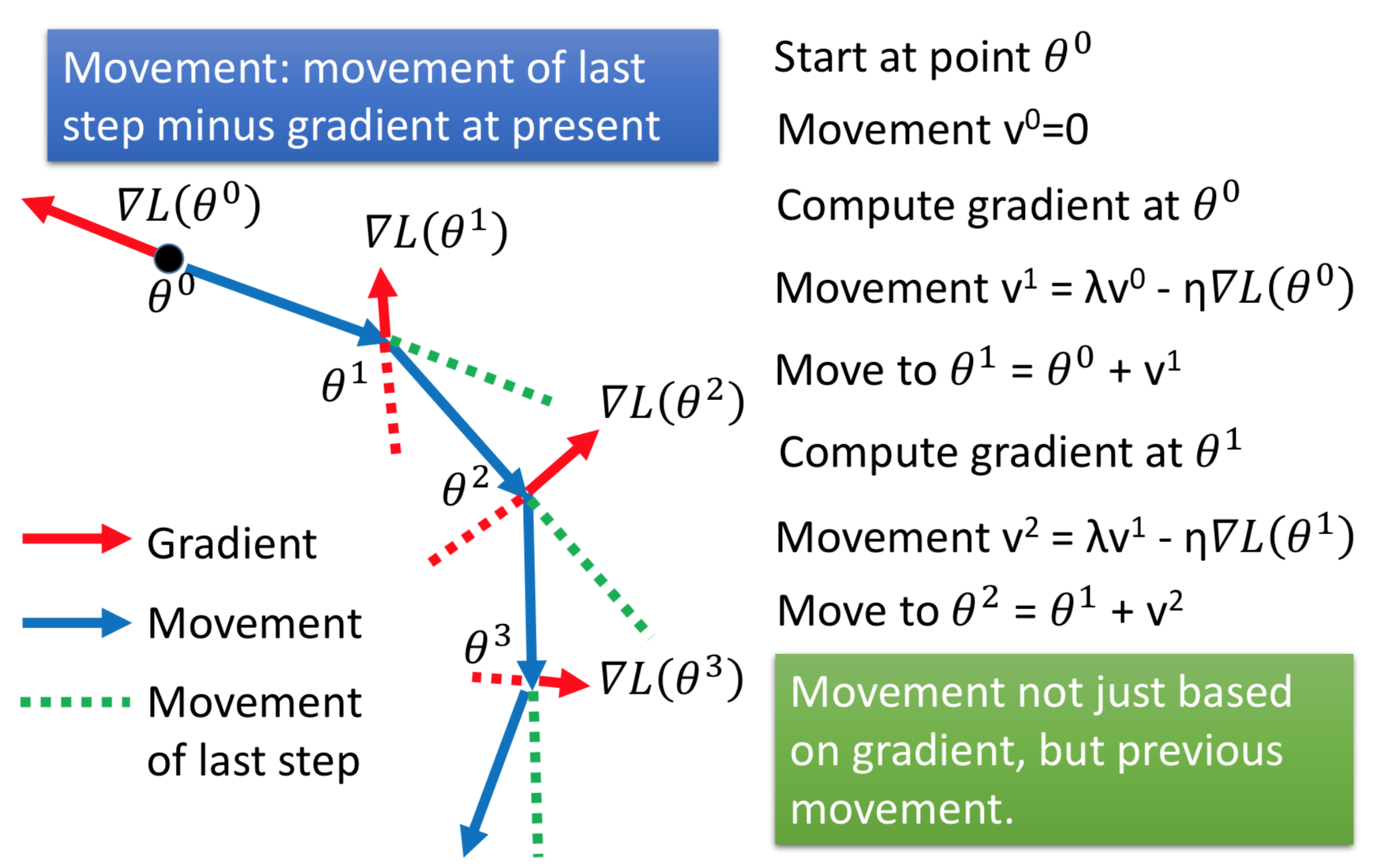$\phantom{\rule{1em}{0ex}}$$\quad$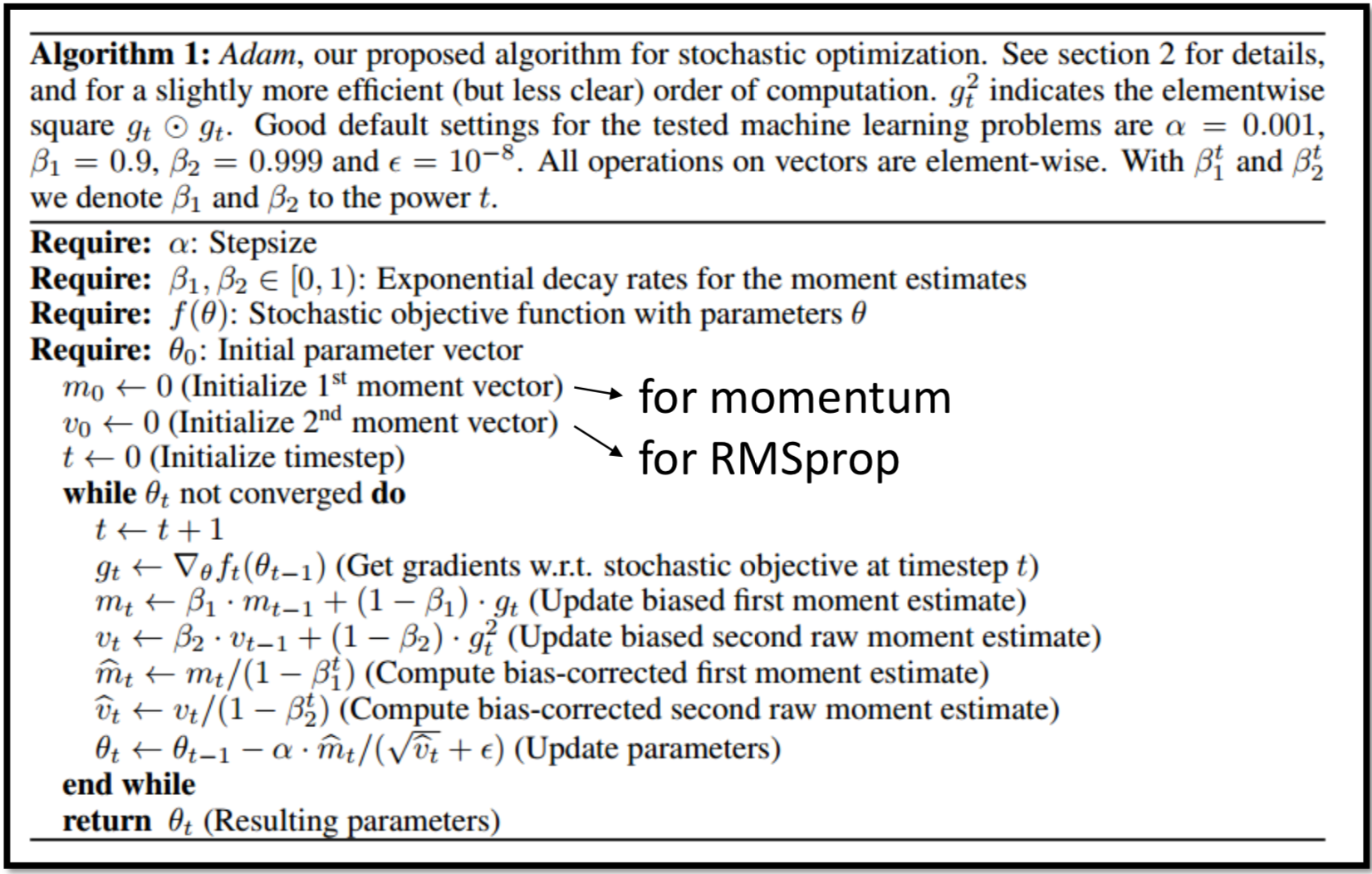$\phantom{\rule{1em}{0ex}}$$\quad$

# Early Stopping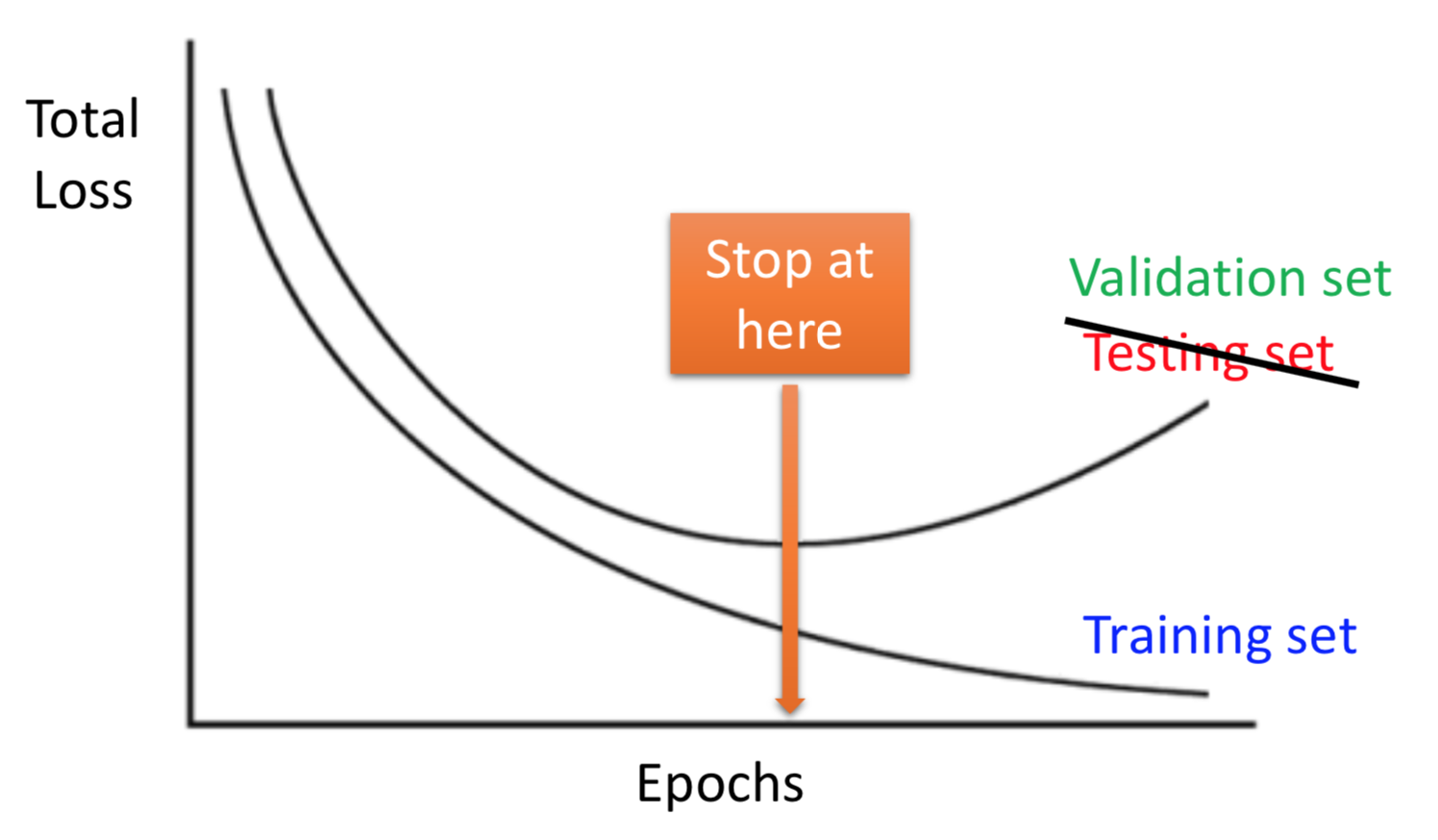$\phantom{\rule{1em}{0ex}}$$\quad$

# Regularization

## L2正则化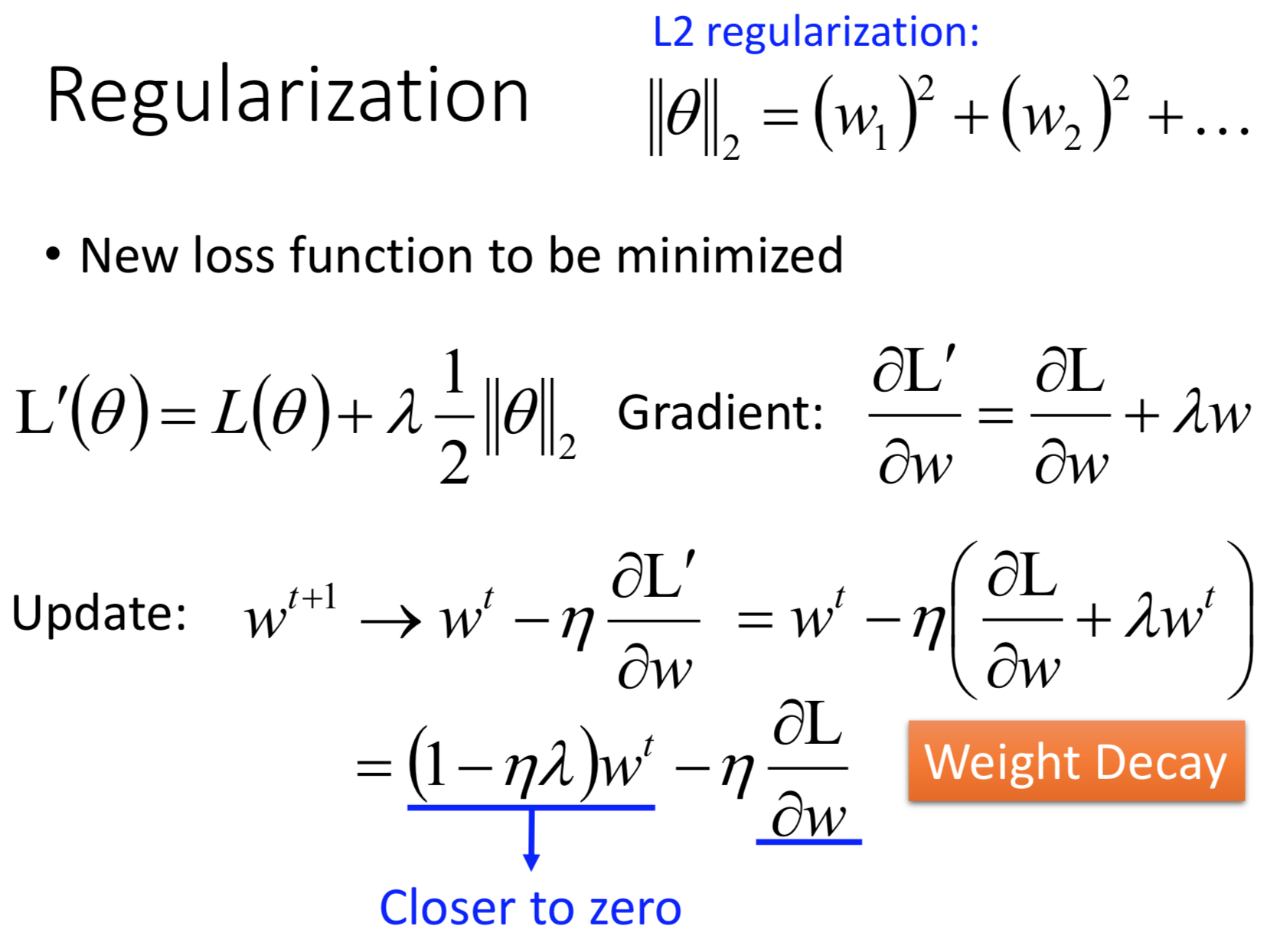$\phantom{\rule{1em}{0ex}}$$\quad$

## L1正则化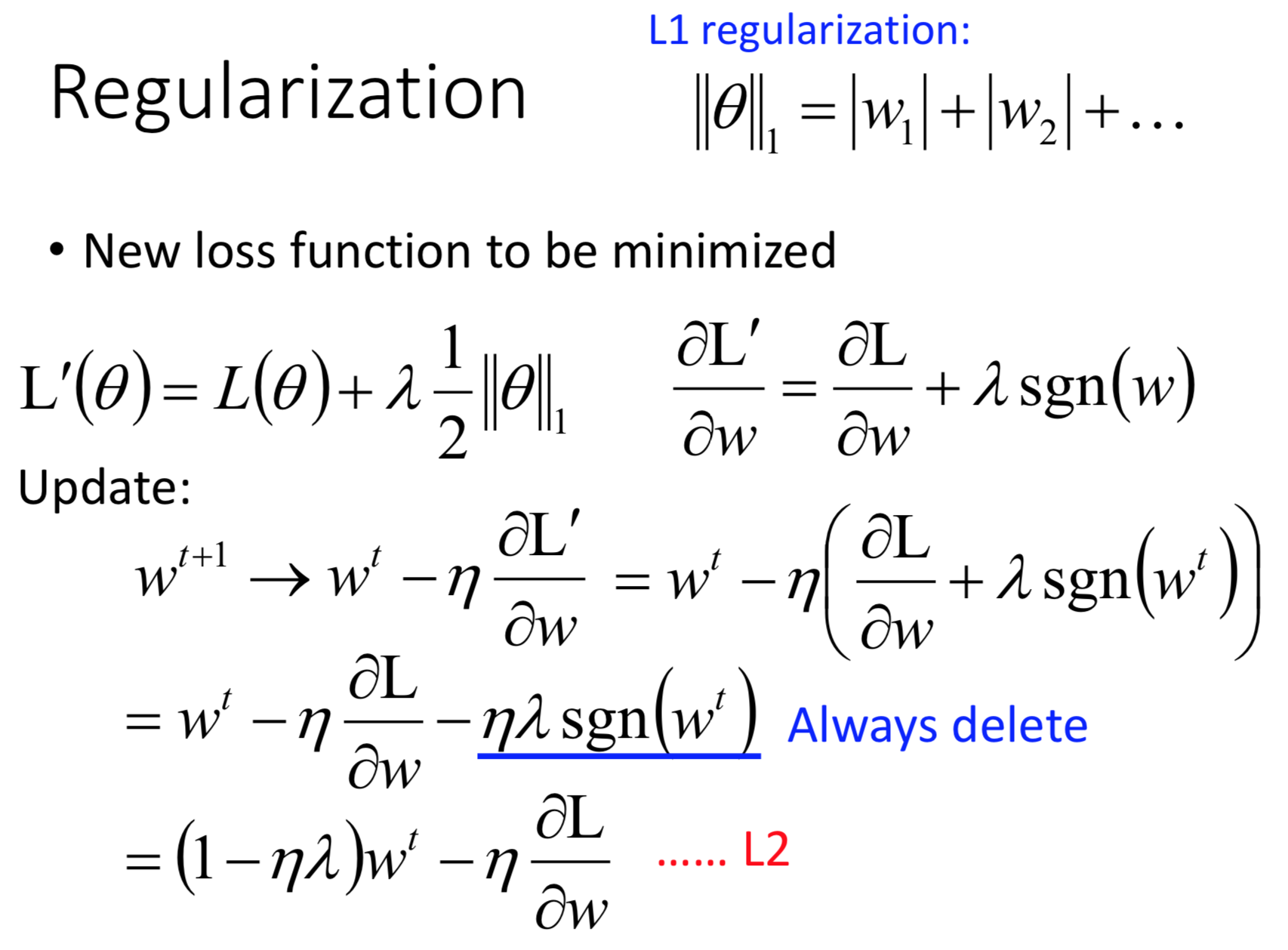$\phantom{\rule{1em}{0ex}}$$\quad$

## L2正则化vsL1正则化

L1和L2相比不同的地方在于二者都让参数接近于0，但是做法不一样：

• L2是乘以一个固定的小于1的值（$1-\eta \lambda$$1-\eta \lambda$），因此L2对比较大的参数的惩罚比较强，L2得到的参数往往聚集于0附近，但不会非常靠近
• L1是减去一个固定的值（$\eta \lambda sgn\left({w}^{t}\right)$$\eta \lambda sgn(w^t)$），L1不管参数大小，惩罚相同，得到的参数差距拉的比较大，0附近的参数更接近于0

$\phantom{\rule{1em}{0ex}}$$\quad$

# Dropout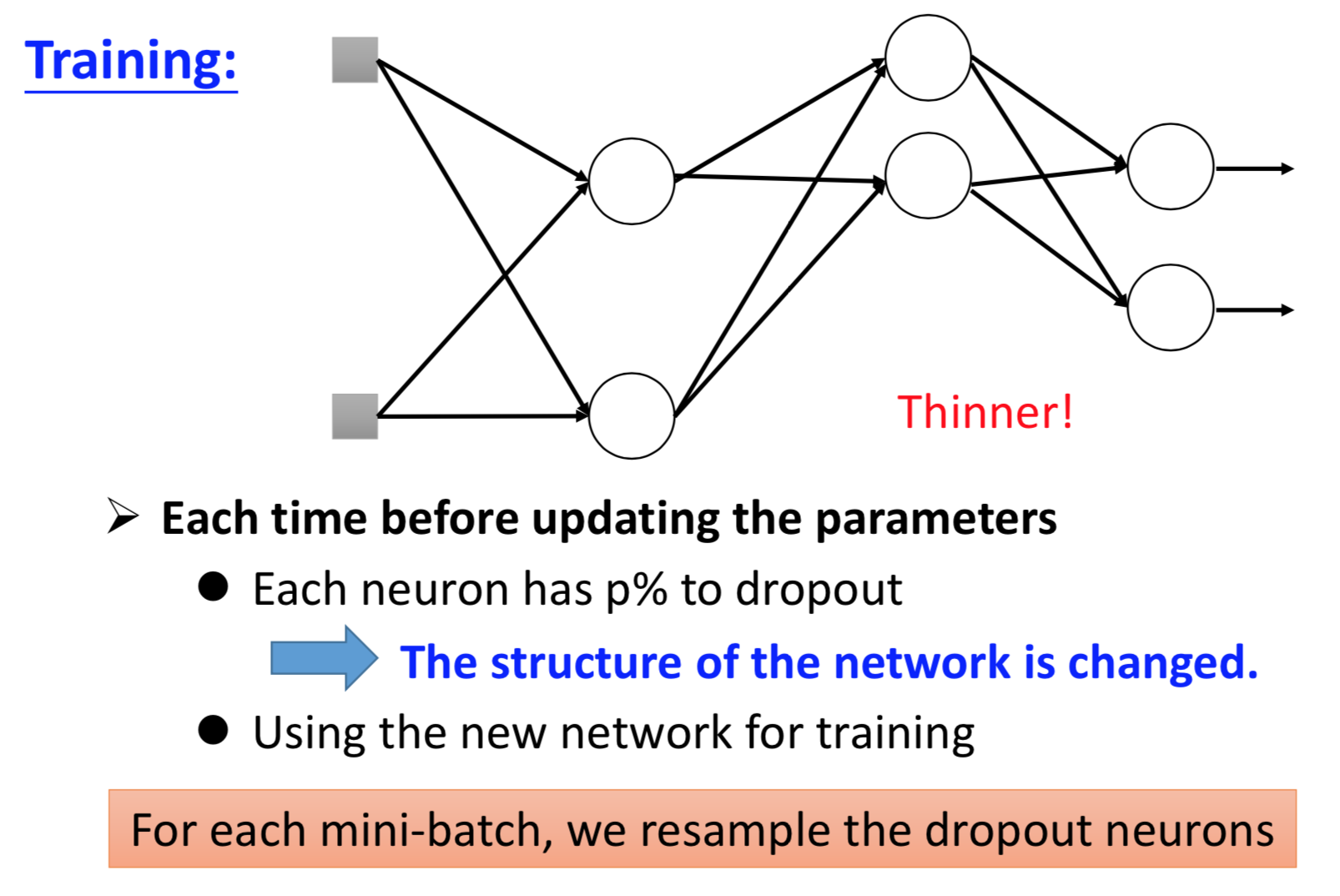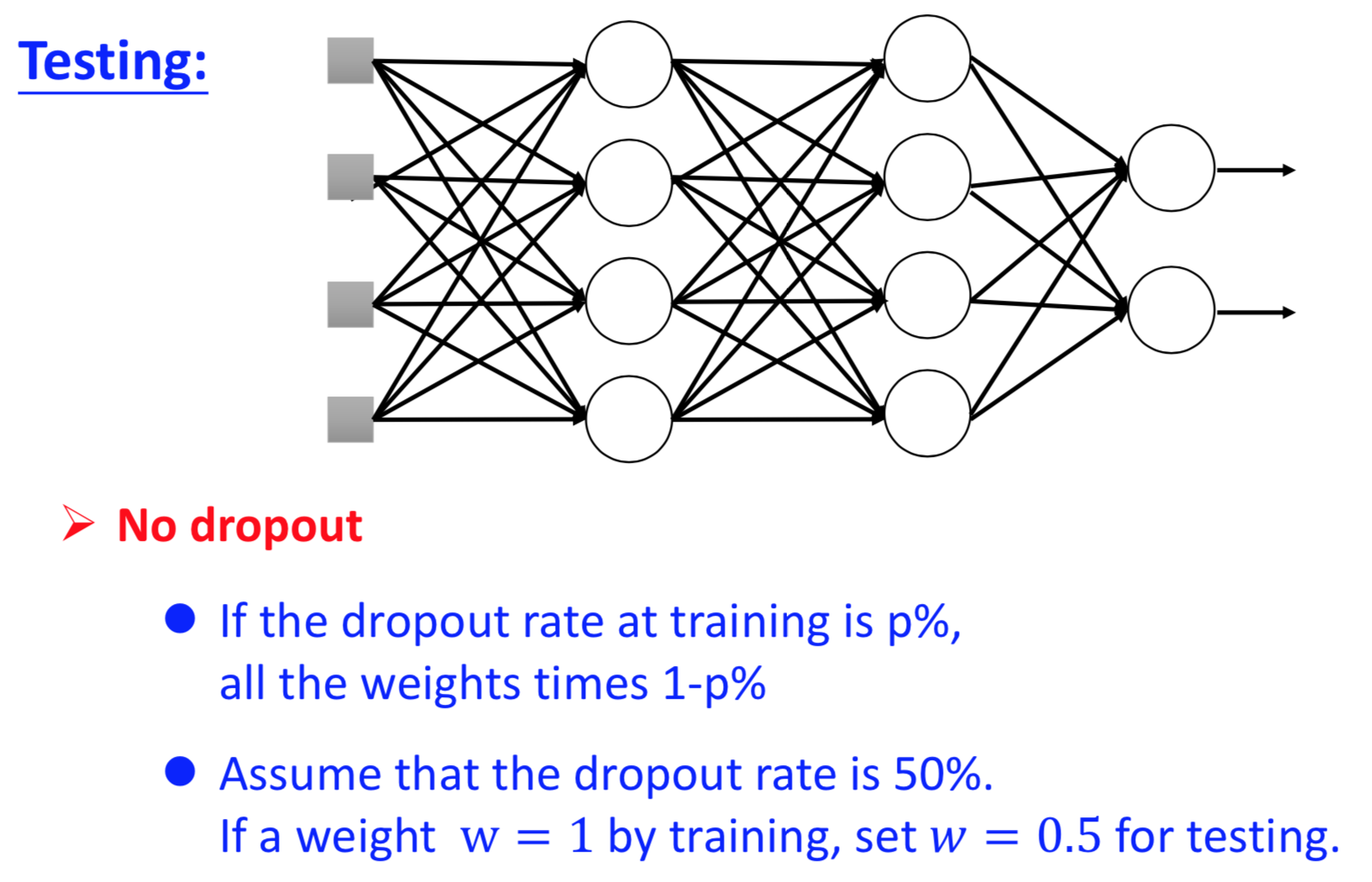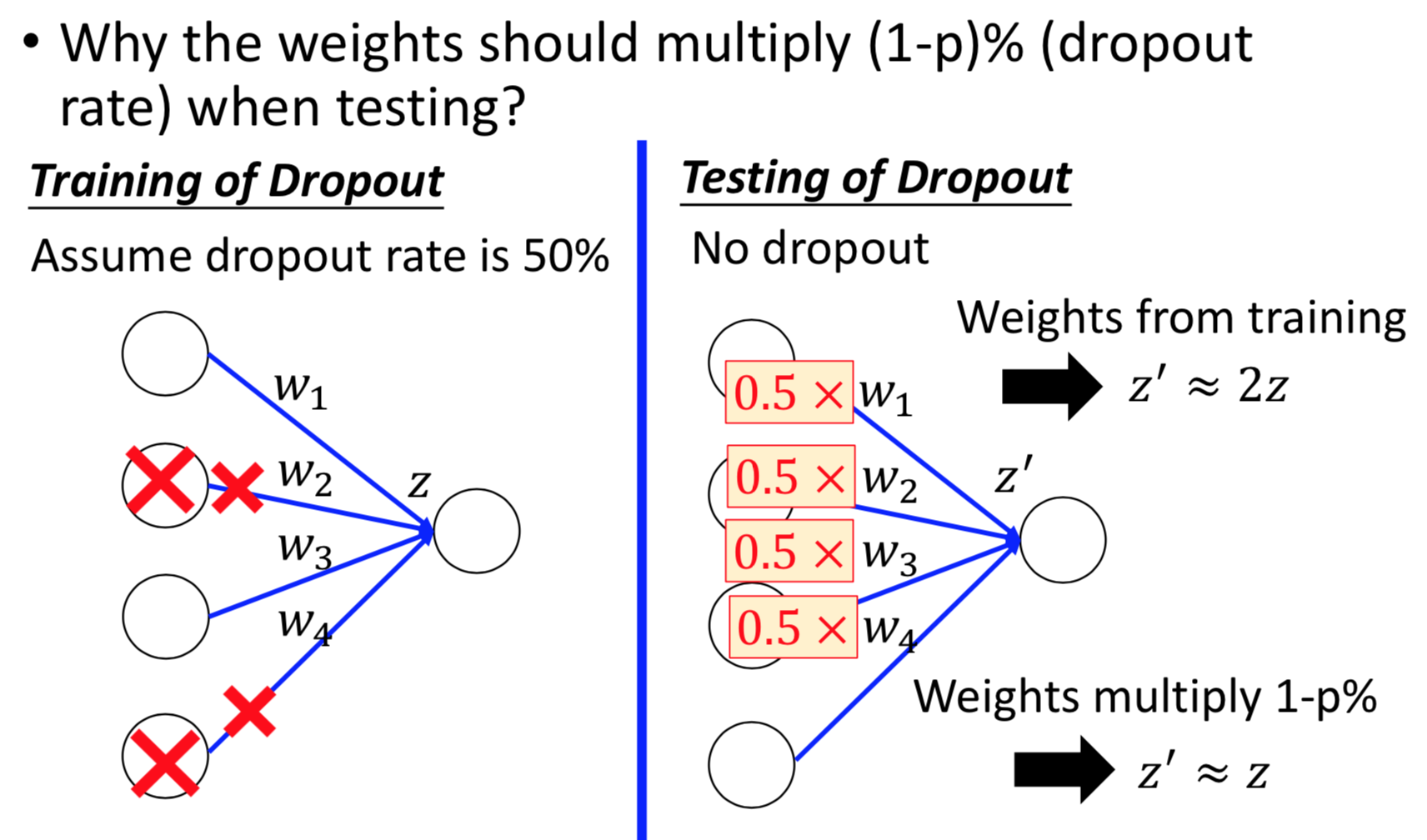Dropout本质是一种ensemble的方法，左右两个模型近似：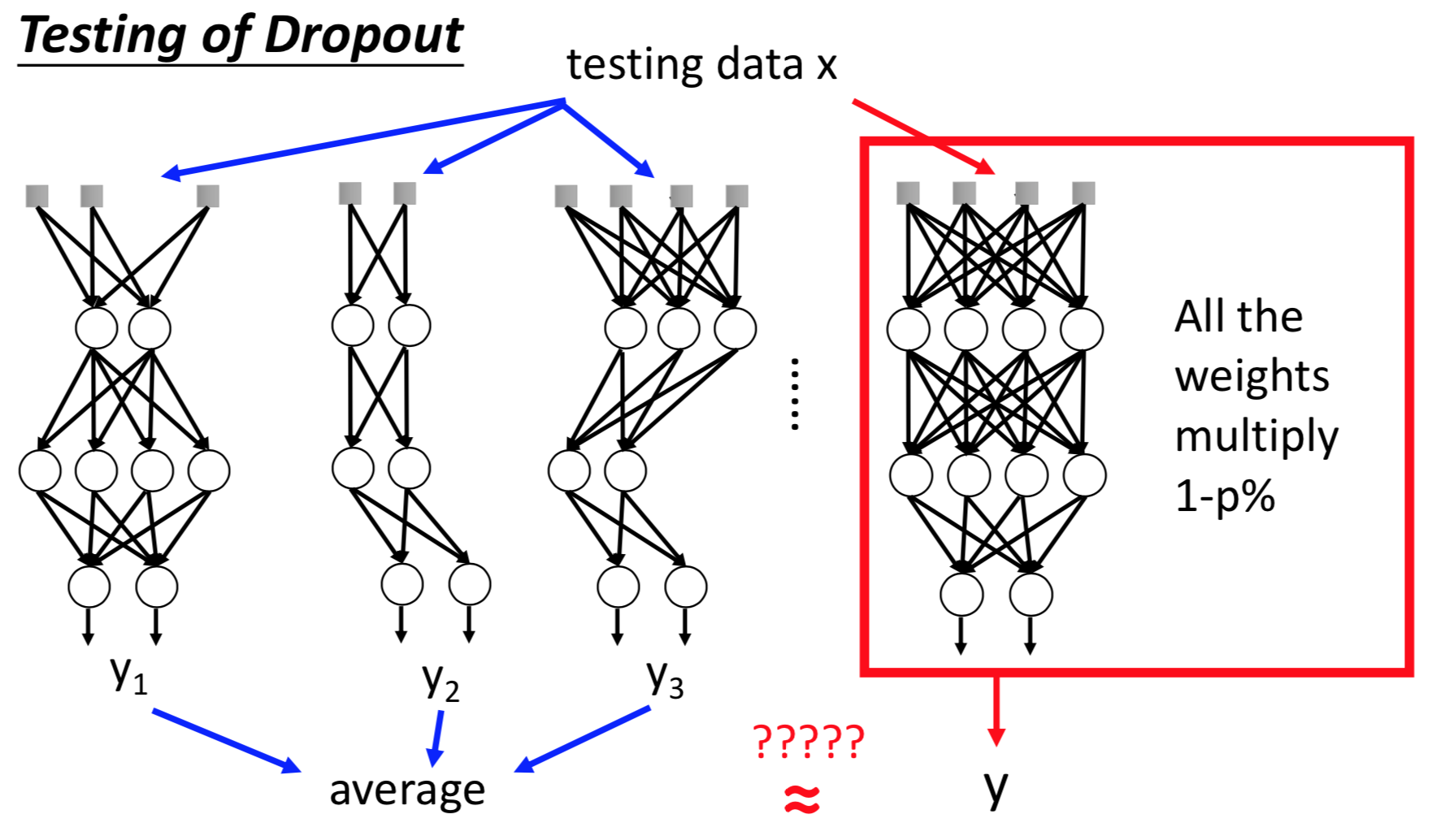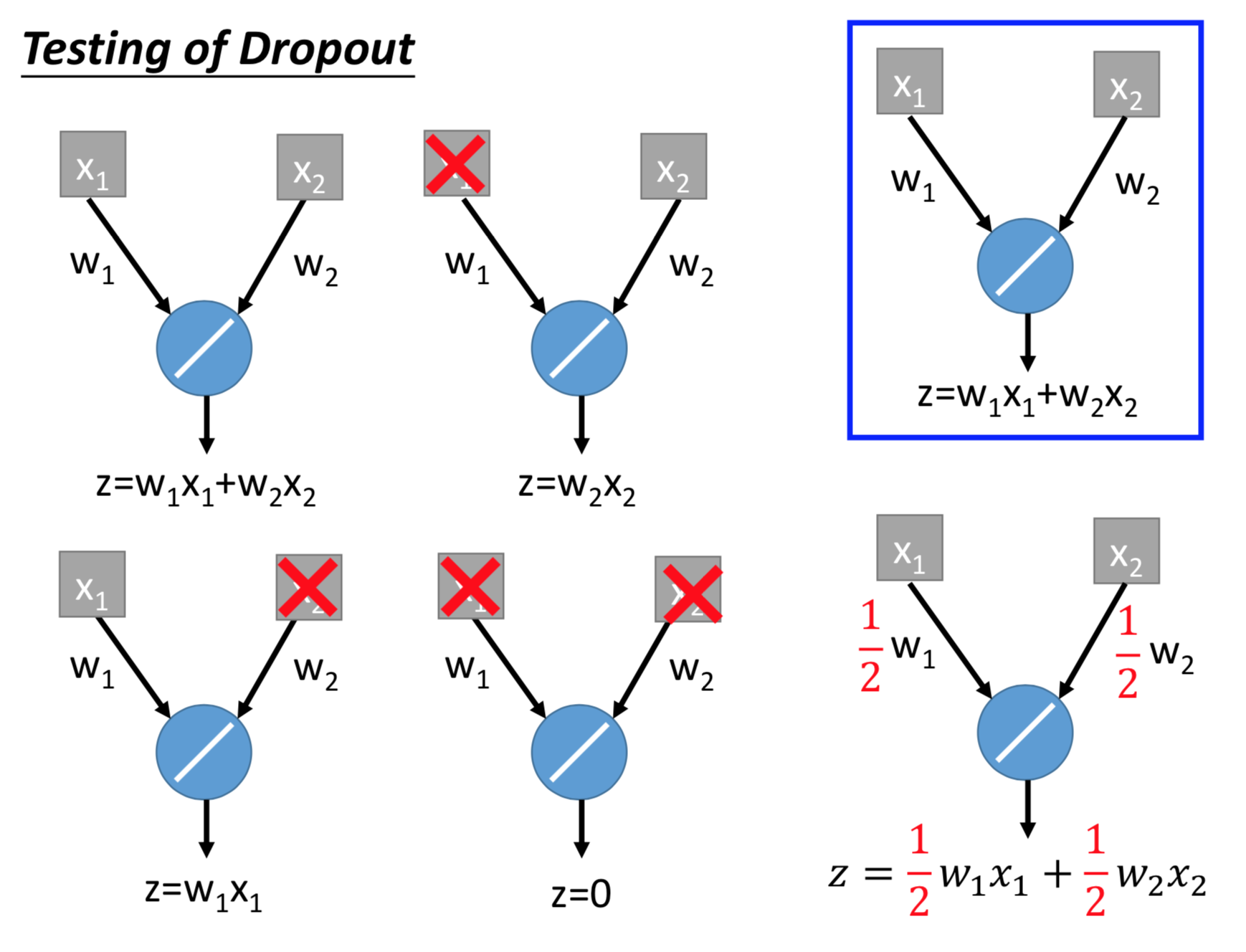©️2019 CSDN 皮肤主题: 技术工厂 设计师: CSDN官方博客